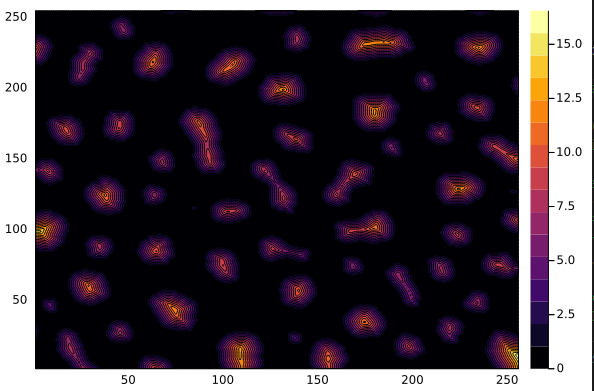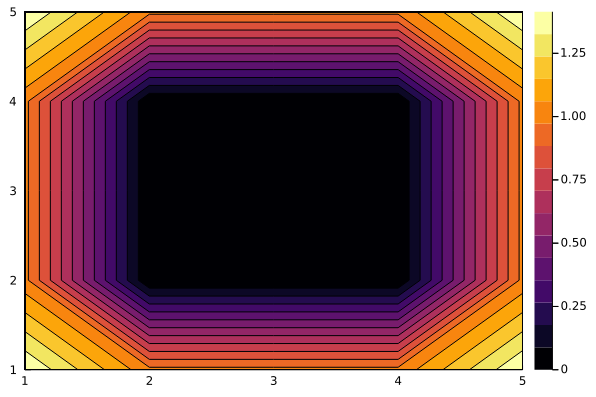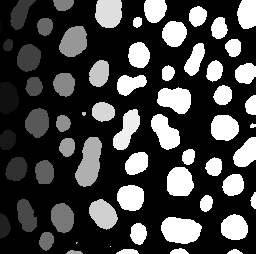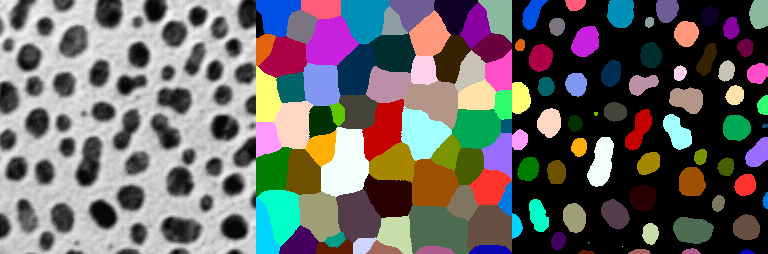# Watershed Segmentation AlgorithmIn this demonstration, we will segment an image using the watershed algorithm and learn how it segments those images. We will using ImageSegmentation.jl which provides implementation of several image segmentation algorithms.

using Images
using ImageSegmentation, TestImages
using IndirectArrays

img = testimage("blobs")
img_example = zeros(Gray, 5, 5)
img_example[2:4,2:4] .=  Gray(0.6)
bw = Gray.(img) .> 0.5
bw_example = img_example .> 0.5
5×5 BitMatrix:
0  0  0  0  0
0  1  1  1  0
0  1  1  1  0
0  1  1  1  0
0  0  0  0  0
bw_transform = feature_transform(bw)
bw_transform_example = feature_transform(bw_example)
5×5 Matrix{CartesianIndex{2}}:
CartesianIndex(2, 2)  CartesianIndex(2, 2)  …  CartesianIndex(2, 4)
CartesianIndex(2, 2)  CartesianIndex(2, 2)     CartesianIndex(2, 4)
CartesianIndex(3, 2)  CartesianIndex(3, 2)     CartesianIndex(3, 4)
CartesianIndex(4, 2)  CartesianIndex(4, 2)     CartesianIndex(4, 4)
CartesianIndex(4, 2)  CartesianIndex(4, 2)     CartesianIndex(4, 4)

feature_transform allows us to find feature transform of a binary image(bw) , it finds the closest "feature" (positions where bw is true) for each location in bw. Specifically, F[i] is a CartesianIndex encoding the position closest to i for which bw[F[i]] is true. In cases where two or more features in bw have the same distance from i, an arbitrary feature is chosen. If bw has no true values, then all locations are mapped to an index where each coordinate is typemin(Int).

For example, the closest true to bw_example[1,1] exists at CartesianIndex(2, 2), hence it's assigned CartesianIndex(2, 2). For other positions in bw_example it is processed similarly.

dist = 1 .- distance_transform(bw_transform)
dist_example = 1 .- distance_transform(bw_transform_example)
5×5 Matrix{Float64}:
-0.414214  0.0  0.0  0.0  -0.414214
0.0       1.0  1.0  1.0   0.0
0.0       1.0  1.0  1.0   0.0
0.0       1.0  1.0  1.0   0.0
-0.414214  0.0  0.0  0.0  -0.414214
Dist(distance transform for img)Dist(distance transform for img_example)distance transform of bw_transform where each element in the array each element F[i] represents a "target" or "feature" location assigned to i. Specifically, D[i] is the distance between i and F[i]. Optionally specify the weight w assigned to each coordinate; the default value of nothing is equivalent to w=(1,1,...).

In bw_transform, element at [1,1] has CartesianIndex(2, 2) in its place and D[i] for this will be distance between CartesianIndex(1, 1) and CartesianIndex(2, 2) which is sqrt(2).

dist_trans = dist .< 1
markers = label_components(dist_trans)
markers_example = label_components(dist_example .< 0.5)
Gray.(markers/32.0) # each of the blobs is slightly differently marked by label_components from 1 to 64label_components finds the connected components in a binary array dist_trans. You can provide a list indicating which dimensions are used to determine connectivity. For example, region = [1,3] would not test neighbors along dimension 2 for connectivity. This corresponds to just the nearest neighbors, i.e., 4-connectivity in 2d and 6-connectivity in 3d. The default is region = 1:ndims(A). The output label is an integer array, where 0 is used for background pixels, and each connected region gets a different integer index.

segments = watershed(dist, markers)
segments_example = watershed(dist_example , markers_example)
Segmented Image with:
labels map: 5×5 Matrix{Int64}
number of labels: 1

watershed method segments the image using watershed transform. Each basin formed by watershed transform corresponds to a segment. To get segments we provide dist and markers with each region's marker assigned a index starting from 1. Zero means not a marker. If two markers have the same index, their regions will be merged into a single region.

labels = labels_map(segments)
colored_labels = IndirectArray(labels, distinguishable_colors(maximum(labels)))
masked_colored_labels = colored_labels .* (1 .- bw)
mosaic(img, colored_labels, masked_colored_labels; nrow=1)Here we use IndirectArray to store the indexed image, for more explanation on it please check the tutorial Indexed image in 5 minutes.